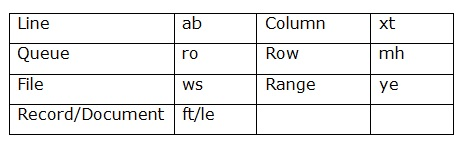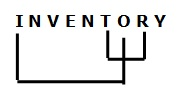# SBI Clerk Prelims Reasoning Ability Questions 2021 (Day-06)

Dear Aspirants, Our IBPS Guide team is providing new series of Reasoning Questions for SBI Clerk Prelims 2021 so the aspirants can practice it on a daily basis. These questions are framed by our skilled experts after understanding your needs thoroughly. Aspirants can practice these new series questions daily to familiarize with the exact exam pattern and make your preparation effective.

Start Quiz

Coding Decoding

Direction (1-4): Study the following information carefully and answer the below questions.

In a certain code language

‘Line Column Queue row’ means ‘ab mh ro xt’,

‘Row File Queue Range’ means ‘ro ye ws mh’,

‘File Document Record Row’ means ‘ws mh ft le’,

‘Line Record Document Range’ means ‘le ye ft ab’

1) What does “ro” represent in a code language?

A.Queue

B.Column

C.Row

D.Line

E.None of these

2) What is the code for ‘Column File’ in the given language?

A.xt le

B.ws ab

C.ro mh

D.xt ws

E.None of these

3) What is the code for ‘Document’ in the given language?

A.ft

B.le

C.ye

D.ab

E.Either a or b

4) What does “ab ft mh” represent in a code language?

A.File Record column

B.Line Record Row

C.Row Line Document

D.Line Queue Row

E.Either b or c

Misc

5) How many pairs of letters are there in the word “INVENTORY” each of which has as many letters between them in the word as in the English alphabetical series?

A.One

B.Two

C.Four

D.None

E.Three

Alpha Numeric Series

Direction (6-10): Study the following information carefully and answer the questions given below:

L Y € L 1 2 K ≤ 5 G € 7 N β C ± T × 2 Ω 6 ∞ 2 £ 3 A V B O ¥ © 2 4 G P 1 X T

6) Which of the following is the fifth to the left of the seventeenth element from the left end of the above arrangement?

A.N

B.7

C.G

D.€

E

7) Which of the following is exactly in the middle between N and 3 in the above arrangement?

A.T

B.2

C

D.Ω

E.6

8) Four of the following five are alike in a certain way based on their position in the above arrangement and so form a group. Which is the one that does not belong to that group?

A.LK2

B.N±C

C.3BV

D.GT1

E.2A3

9) How many such symbols are there in the above arrangement each of which is immediately preceded by a number and immediately followed by a consonant?

A.5

B.2

C.1

D.3

E.None

10) How many 2’s are given in this arrangement which is immediately followed by a symbol?

A.1

B.2

C.3

D.4

E.None

Directions (1-4):